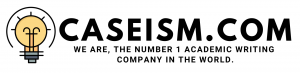# DuPont Corporation: Sale of Performance Coatings Case Solution & Answer

Working from case Exhibit 9, estimate the increase in value a PE sponsor can obtain assuming (a,b,c,d).

DuPont has no debt in its capital structure currently therefore; the valuation for the Performance Coatings division in exhibit 9 has been performed by calculating the UN levered free cash flows and then discounting them to the cost of equity of 11.20% of DuPont.

However, in order to determine the increase in the value, which could be obtained by the private equity sponsors by investing in the Performance Coatings division, the valuation for this division has been performed once again as stated in parts a-d.

Including these set of assumptions, other assumptions which have also been used in the valuation of the DPC division are that the working capital investment is assumed to remain at the same 15% of sales level; the investment in capital expenditures would also be same as in exhibit 9.

The cost of debt for valuing the unlevered free cash flows is same which is 11.20% and the cost of debt taken for valuation of tax savings is the blended cost of debt of 6.75%. Lastly, since depreciation and amortization is a non-cash expense therefore, it has been added back to calculate the final UN levered free cash flows.

Based on these set of assumptions the UN levered free cash flows have been calculated for the DPC division as shown in excel spreadsheet. The terminal value has been calculated as the product of 7.5x of the projected 2016 EBITDA is about \$ 682 million. This gives us a terminal value at the time of exit by the PE sponsors of \$ 5.4 billion.

In order to calculate the present value of the tax shield, first of all the amount of the debt required for financing this LBO transaction has been calculated. The amount of the debt raised is equal to 5x of the trailing EBITDA for the next 12 months which has been calculated as \$ 565 million.

This gives a total level of debt for financing this LBO transaction of \$ 2.83 billion approximately with a payment of \$ 351.21 million made in each period.

Finally, the loan amortization schedule has been created for the investment horizon which gives us he interest due at the end of each period. Based upon a tax rate of 25% as provided in exhibit 9, the tax savings have been calculated. After that they are discounted at the blended cost of debt of 6.75%, the present value of tax shield has been calculated which is \$ 174.63 million total.

The enterprise value of DPC is \$ 4.316 billion which is the sum of the present value of tax shield, terminal value, and unlevered free cash flows of \$ 174.63 million, \$ 5.4 billion and 4.142 billion respectively.

Value Increase for Private Equity Sponsor

The value created for the private equity sponsors from this transaction would simply be the difference between the values of the investment at the start and at the end of the period. The purchase price for this investment is based on a purchase price/EBITDA multiple of 9x. Furthermore, the value of DPC division has also been calculated as shown in excel spreadsheet at the end of the investment horizon in 2016.

Based on the purchase price/EBITDA multiple of 9x, the purchase price for DPC has been calculated to be \$ 3.348 billion and the price for the division at the end of the investment horizon in the year 2016 is \$ 5.457 billion. The total multiple arbitrage value created by the private equity sponsors would be therefore, equal to \$ 2.12 billion. In percentage terms, the value created by the private equity sponsors over their initial purchase price is 39%. ………….

This is just a sample partial work. Please place the order on the website to get your own originally done case solution